# Triangle - 7th grade (12y) - math problems

#### Number of problems found: 208

• Perimeter of a triangle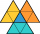If the perimeter of a triangle is 6 2/3 cm and the lengths of two of its sides are 2 1/2 cm and 3 1/3 cm, find the length if the third side
• The two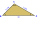The two angles of a triangle are 78° and 82°. So what is the measure of the remaining third angle?
• Diamond PQOR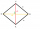In the diamond PQOR, the diagonal RQ is 4 cm long and the angle RPQ is 60°. What is the circumference of this diamond?
• JoanneJoanne and Roger are planting a rectangular garden. The garden is 8 1/2 ft by 13 ft. They want to use half of the garden for cucumbers and half of the garden for tomatoes. They decide to separate the garden into two right triangles. What is the area of th
• Main/central vertex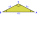ABC is an isosceles triangle with base BC and main vertex A. The angle at vertex A is 18°. What will be the size of the angle at vertex B?
• Find theFind the third interior angle of the triangle ABC where: α = 48°, γ = 65°.
• Divide an isosceles triangleHow to divide an isosceles triangle into two parts with equal contents perpendicular to the axis of symmetry (into a trapezoid and a triangle)?
• A triangle 2A triangle has a base of 2 inches and a height of 2 3/4 inches. What is the area of the triangle?
• MO Z7–I–6 2021In the triangle ABC, point D lies on the AC side and point E on the BC side. The sizes of the angles ABD, BAE, CAE and CBD are 30°, 60°, 20° and 30°, respectively. Find the size of the AED angle.
• The perimeter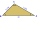The triangle has one side 5 cm long and the another 11 cm long. What can be the smallest and what is the largest perimeter?
• Gamma angleFind the magnitude of the gamma angle in triangle ABC if: α = 38° 56 ’and β = 47° 54’.
• Prism height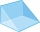What is the prism's height with the base of a right triangle of 6 cm and 9 cm? The diaphragm is 10.8 cm long. The volume of the prism is 58 cm3. Calculate its surface.
• Inner anglesThe magnitude of the internal angle at the main vertex C of the isosceles triangle ABC is 72°. The line p, parallel to the base of this triangle, divides the triangle into a trapezoid and a smaller triangle. How big are the inner angles of the trapezoid?
• GardensThe area of the square garden is 3/4 of the area of the triangular garden with sides of 80 m, 50 m, 50 m. How many meters of the fence do we need to fence a square garden?
• A cliff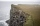A line from the top of a cliff to the ground passes just over the top of a pole 5 ft high and meets the ground at a point 8 ft from the base of the pole. If the point is 93 ft from the base of the​ cliff, how high is the​ cliff?
• Trapezoid - constructionConstruct a trapezoid KLMN, where: k = 9 cm, l = 4 cm, m = 5 cm and angle α = 45°
• Hexagonal prism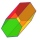Calculate the volume and surface of a regular hexagonal prism with the edge of the base a = 6 cm with the corresponding height v1 = 5.2cm and the height of the prism h = 1 dm.The nine-meter poplar casts a shadow 16.2 m long. How long does a shadow cast by Peter at the same time, if it is 1.4 m high?
• Height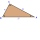The content of the triangle is 35 cm2. The length of the base is 10 cm. Determine the length of the height on the base.
• Chimney and treeCalculate the height of the factory chimney, which casts a shadow 6.5 m long in the afternoon. At the same time, a 6 m high tree standing near it casts a shadow 25 dm long.

Do you have an exciting math question or word problem that you can't solve? Ask a question or post a math problem, and we can try to solve it.

We will send a solution to your e-mail address. Solved examples are also published here. Please enter the e-mail correctly and check whether you don't have a full mailbox.